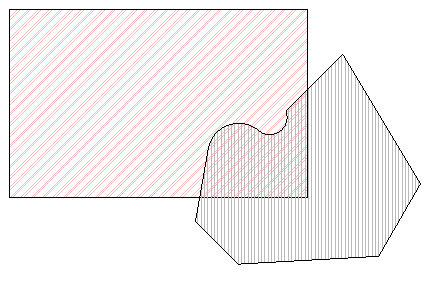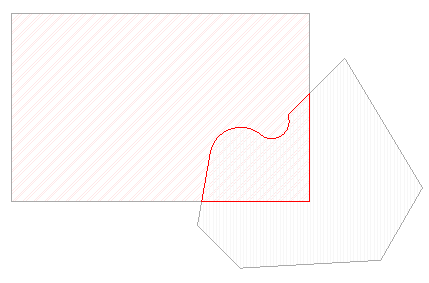### Boolean Operations for 2D Polygons

Friday the thirteenth! What has gone wrong so far today? Keeping my fingers crossed ...

We recently discussed the issue of determining the wall area adjacent to a room and mentioned the fact that the simplest way to calculate such an overlapping area might be the use of a free-standing library for performing Boolean set operations on two-dimensional polygons. As said, there are many such libraries available, as you can see by googling for "polygon boolean" or by looking at the Wikipedia article on Boolean operations on polygons. I suggested possibly using the Generic Polygon Clipper (gpc) and its C# wrapper GpcWrapper. Now my colleague Adam Nagy – the pronunciation is 'nadj', according to Adam, and an interesting topic in itself – went ahead and wrote a sample application demonstrating the use of this tool in a Revit add-in.

Adam's sample application is named GpcNET. It defines an external command within the namespace GpcNET. It also includes the GpcWrapper code in the same namespace, so that all its classes are immediately available to the command class.

You need to select two floors before running the command. If not, it will prompt you to do so and abort. The two floors are used to define a two-dimensional polygon each. The intersection of the two polygons is computed. If the result is a valid polygon, a new third floor is created. It uses the intersection result to define its profile CurveArray, and the same floor type and level as the first selected floor.

Here is the code that extracts the profile from a selected floor and creates a GPC polygon object from it:

```Polygon getPolygon( Floor floor )
{
Options geomOptions
= app.Create.NewGeometryOptions();

GeoElement elem
= floor.get_Geometry( geomOptions );

List<Vertex> vertices = new List<Vertex>();

foreach( object obj in elem.Objects )
{
Solid solid = obj as Solid;
if( null != solid )
{
Face face = solid.Faces.get_Item( 0 );
EdgeArray loop = face.EdgeLoops.get_Item( 0 );
foreach( Edge edge in loop )
{
XYZArray edgePts = edge.Tessellate();
int n = edgePts.Size;
for( int i = 0; i < n - 1; ++i )
{
XYZ p = edgePts.get_Item( i );
vertices.Add( new Vertex( p.X, p.Y ) );
}
}
break;
}
}
VertexList vertexList = new VertexList();
vertexList.NofVertices = vertices.Count;
vertexList.Vertex = vertices.ToArray();
Polygon poly = new Polygon();
poly.AddContour( vertexList, false );
return poly;
}
```

Here is the code for the Execute method of the command, i.e. the command mainline:

```CmdResult rc = CmdResult.Failed;
app = commandData.Application;
doc = app.ActiveDocument;

Floor[] floors = new Floor { null, null };

// get the first 2 floors of the selection
foreach( RvtElement e in doc.Selection.Elements )
{
if( null == floors )
{
floors = e as Floor;
}
else if( null == floors )
{
floors = e as Floor;
}
else
{
break;
}
}

// if the selction did not contain two floors, return
if( null == floors || null == floors )
{
MessageBox.Show(
"Please select two floors before"
+ " running this command.",
"GpcNET" );
}
else
{
// get the intersection
Polygon poly1 = getPolygon( floors );

Polygon poly2 = getPolygon( floors );

Polygon poly3 = poly1.Clip(
GpcOperation.Intersection,
poly2 );

// if it looks like a valid polygon, create a new floor
if( 0 < poly3.NofContours )
{
CurveArray curves = app.Create.NewCurveArray();
VertexList v = poly3.Contour;
int i, j, n = v.NofVertices;
for( i = 0; i < n; ++i )
{
j = ( i + 1 ) % n;
Vertex p = v.Vertex[i];
Vertex q = v.Vertex[j];

curves.Append( app.Create.NewLineBound(
app.Create.NewXYZ( p.X, p.Y, 0 ),
app.Create.NewXYZ( q.X, q.Y, 0 ) ) );
}
doc.Create.NewFloor( curves,
floors.FloorType,
floors.Level, false );

rc = CmdResult.Succeeded;
}
}
return rc;
```

A short readme file and sample Revit.ini snippet are included with the project. Here are the steps to rebuild and install it:

1. In the Visual Studio project, adjust the Properties > Build > Output Path to point to the Revit.exe folder, e.g. "C:\Program Files\Revit Architecture 2009\Program".
2. Compile the project.
3. Copy the gpc.dll file from the project folder to the Revit.exe folder.
4. Adjust your Revit.ini file, for instance using the text in the sample Revit.ini file provided.

Please note that the GPC library is only free to use for non-commercial use. Please contact the vendor if you are thinking of using it in a commercial product.

The zip file includes a sample project named floors.rvt containing two floors. Here is what they look like before running the command:Here is the third floor created using the profile resulting from the intersection of the two, which have been temporarily half-toned:To apply these results to the problem we started the discussion with, the wall area adjacent to a given room, we would need to transform the vertical wall and room faces into 2D, as described in the discussion on polygon transformation.

Here is the complete Visual Studio GpcNET solution.